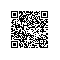# C++程序设计：原理与实践（进阶篇）16.5　数值算法

16.5　数值算法

x = accumulate(b,e,i) 累加序列中的值；例如，对{a, b, c, d}计算i+a+b+c+d。结果x的类型与初始值i的类型一致

x = inner_product(b,e,b2,i) 将两个序列的对应元素相乘并将结果累加。例如，对{a, b, c, d}和{e, f, g, h}计算i+a·e+b·f+c·g+d·h。结果x的类型与初始值i的类型一致

r = partial_sum(b,e,r) 对一个序列的前n个元素进行累加，并将累加结果生成一个序列。例如，对{a, b, c, d}将生成{a, a+b, a+b+c, a+b+c+d}

r = adjacent_difference(b,e,b2,r) 对一个序列的相邻元素进行减操作，并将得到的差生成一个序列。例如，对{a, b, c, d}将生成{a, b-a, c-b, d-c}

16.5.1　累积

accumulate()是最简单但最有用的数值算法。在其最简单的形式中，该算法将一个序列中的值进行累加：

16.5.2　泛化accumulate()

16.5.3　内积

16.5.4　泛化inner_product()

inner_product()可以像accumulate()那样泛化，但它需要两个额外参数：一个用于将累加器与新值组合起来（与accumulate()完全一样），另一个用于组合元素值对：